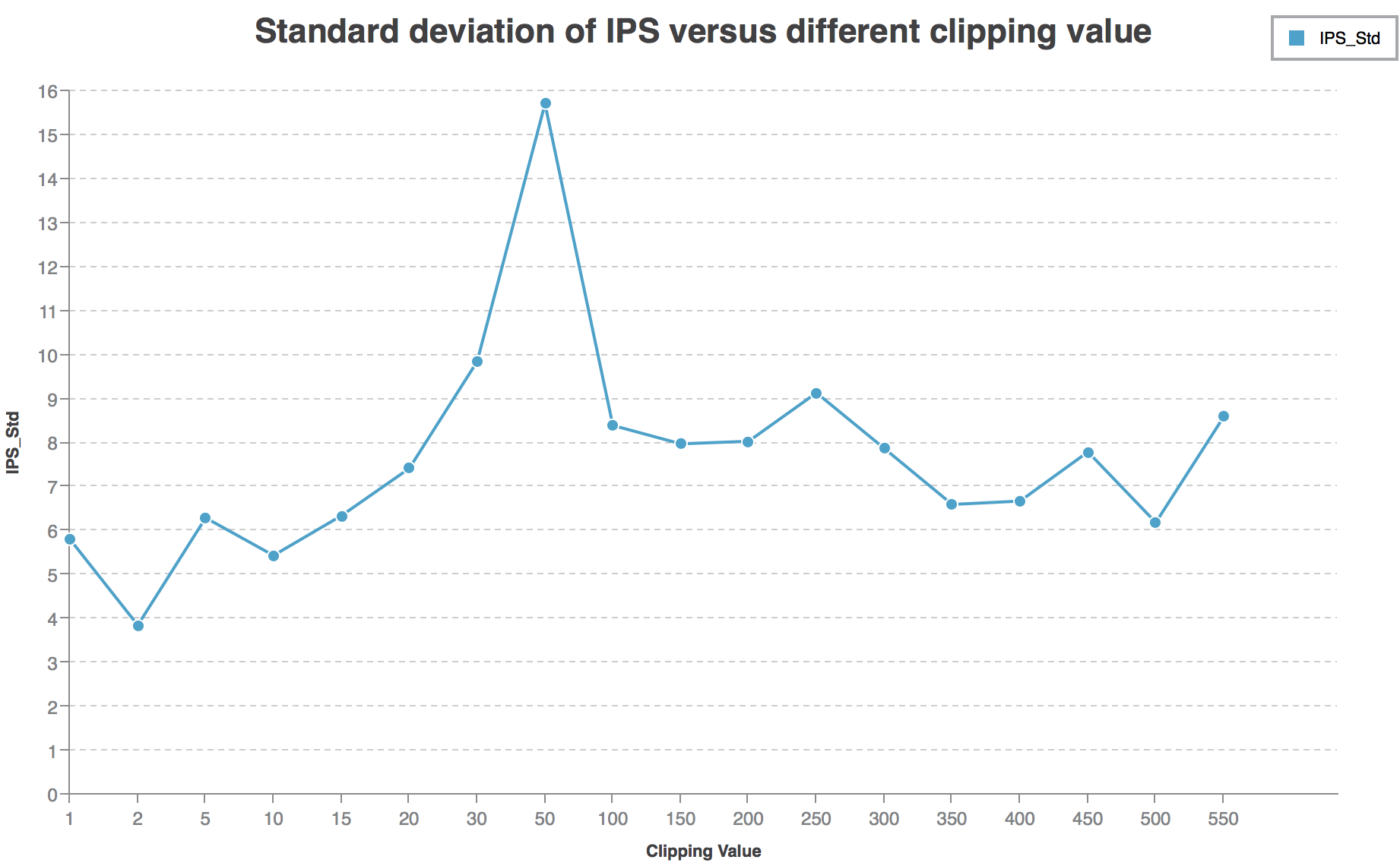Weekly ReportJinning Li Aug 07, 2018

Weekly Report 

Jinning, 08/07/2018

Clip experiment on large dataset

Got the result of Clipping with $\min\{\frac{1}{p}, c\}$. This result is trained and tested on large dataset.

Loss function used (weighted BCE loss):

$-\min\{\frac{1}{p}~,~c\}\left[ y \cdot \log \sigma(x) + (1 - y) \cdot \log (1 - \sigma(x)) \right]$

$c\in$[1, 2, 5, 10, 15, 20, 30, 50, 100, 150, 200, 250, 300, 350, 400, 450, 500, 550].

In the training set, the value of $\frac{1}{p}$ varies among $[1, 2917566]$. Its average is $114.57$.

Distribution of inverse propensity

In order to survey the distribution of $\frac{1}{p}$ , the following diagram show the percentage of $\frac{1}{p}$ larger than given $c$ (which is clipped):[100.00%] 14175476 in 14175476 larger than 1
[96.52%] 13682662 in 14175476 larger than 5
[95.78%] 13577934 in 14175476 larger than 10
[56.56%] 8018069 in 14175476 larger than 20
[45.15%] 6400434 in 14175476 larger than 30
[32.50%] 4606483 in 14175476 larger than 50
[18.09%] 2564603 in 14175476 larger than 100
[11.79%] 1671481 in 14175476 larger than 150
[8.55%] 1212462 in 14175476 larger than 200
[6.62%] 938342 in 14175476 larger than 250
[5.35%] 758935 in 14175476 larger than 300
[4.45%] 631364 in 14175476 larger than 350
[3.80%] 538490 in 14175476 larger than 400
[3.30%] 467660 in 14175476 larger than 450
[2.91%] 412324 in 14175476 larger than 500
[2.59%] 367630 in 14175476 larger than 550
[1.26%] 178423 in 14175476 larger than 1000
[0.16%] 23365 in 14175476 larger than 5000
[0.07%] 9505 in 14175476 larger than 10000
...

The curve of IPS and Standard deviation of IPS versus $c$ :

IPS Curve:IPS-Std Curve:Discussions

Not coincidence

I notice that when $30<c<50$, the IPS is the highest (over $60$). However, the standard deviation is also quite high (over $10$). I train the network again when $c=50$, the IPS is still over $60$. So I think the local peak when $30<c<50$ is not a coincidence.

Why standard deviation is so high?

In the evaluation process, IPS is calculated by

$\frac{10^4}{n^+ + 10n^-}\sum\delta\frac{\frac{score(\hat{x})}{\sum score(x_i)}}{\pi_0}$

In another word, we calculate $\pi_w=\frac{score(\hat{x})}{\sum score(x_i)}$.

Assume the output of our network is $\sigma$, then score is calculated by $score=e^{score-\max(score)}$ in the evaluation program.

The standard deviation is measured by

$\frac{2.58\times \sqrt{n}}{n^+ + 10n^-}\times Stderr[\delta \frac{\frac{ score(\hat{x})}{\sum score(x_i)}}{\pi_0}]$

Note that we apply a post-process trick that $\sigma' = \frac{850100}{1+e^{-\sigma +1.1875}}$.

Before post-process $\sigma$:

896678244; 0:0.011302, 1:0.00727101, 2:0.0111319, 3:0.000752336, 4:0.00235881, 5:0.0131616, 6:0.00344201, 7:0.0268872, 8:0.0108119, 9:0.022044, 10:0.0268872

After post-process $\sigma'$:

896678244; 0:200400, 1:199783, 2:200374, 3:198788, 4:199033, 5:200685, 6:199198, 7:202811, 8:200325, 9:202049, 10:202796

Before post-process $\pi_w=\frac{score(\hat{x})}{\sum score(x_i)}$:

896678244; 0:0.984536, 1:0.980575, 2:0.984368, 3:0.974204, 4:0.97577, 5:0.986368, 6:0.976827, 7:1, 8:0.984053, 9:0.995168, 10:1

After post-process $\pi_w=\frac{score(\hat{x})}{\sum score(x_i)}$:

896678244; 0:0, 1:0, 2:0, 3:0, 4:0, 5:0, 6:0, 7:1, 8:0, 9:0, 10:3.05902e-07

So actually our post-processing makes the policy more strict. For example, as shown above, after post-processing, id=896678244 gets a propensity vector $[0, 0, 0, 0, 0, 0, 0, 1, 0, 0, 3.05902e-07]$. So, if 7 is not the selected candidate, $\pi_w$ will be $0$, else $\pi_w$ will be $1$.

If not post-process, the propensity vector will be $[0.985, 0.981, 0.974, 0.976, 0.986, 0.977, 1, 0.984, 0.995, 1]$. Then $\pi_w$ will be almost $\frac{1}{11}$ no matter which candidate is selected.

So it’s easy to understand after post-processing, standard deviation will be much higher.

And it clear that post-processing is necessary. Because if not, the expectation of $\pi_w$ will always be close to $\frac{1}{11}$, no matter how nice the model is.

I think this is a BUG of the evaluation program. It should not use $score=e^{score-\max(score)}$ but something like $score=e^{\frac{score-\max(score)}{\sum score}}$.

Question: Why Standard deviation varies with clipping value? Why higher IPS often accompanies with higher Standard deviation?

Review what I am doing

What I am doing is training a network $\sigma$ to minimize the loss function (If clipping is applied)

$-\min\{\frac{1}{p}, c\}\left[ y \cdot \log \sigma(x) + (1 - y) \cdot \log (1 - \sigma(x)) \right],$

An important point is that $y$ is whether this Ad is clicked, instead of whether this Ad is chosen to display. This is the difference between banditNet and my net.

Then, we use our network $\sigma_{\bar{w}}$ to estimate the probability of clicking of a given Ad, and use this propensity as the propensity to display this Ad.

This is based on an assumption that $\pi_0$ will always choose Ads with high CTR (reward) to display.

If this assumption is true (of course true), then the IPS:

$IPS = \frac{10^4}{n^+ + 10n^-}\sum\delta\frac{\frac{score(\hat{x})}{\sum score(x_i)}}{\pi_0}$

will be maximized. This is how my net works.

Question: Why do we need to add $\min\{\frac{1}{p}, c\}$ before our BCE loss fuction? Why will this contribute to CTR prediction?

In the banditNet settings, the network will directly calculate propensity for each candidate, or $\pi_w$, then directly maximize the IPS / SNIPS or minimize its Lagrangian.

$IPS = \frac{1}{n}\sum\delta\frac{\pi_w}{\pi_0}$

What’s the best clipping value?

According to the diagram drawn in the beginning, the best clipping value is around $30$ ~ $50$. The percentage of clipped propensities are about $30\%$ ~ $45\%$.

Question: Will $30\%$ ~ $45\%$ always be the best percentage? How to find the relationship between the best clipping value and the distribution of propensity?

Question: Is it true that the more propensities are clipped, the larger bias is and the smaller variance is? What’s the relation between bias-variance-tradeoff and clipping method?

Question: Is there better way to find the best clipping value without grid search?